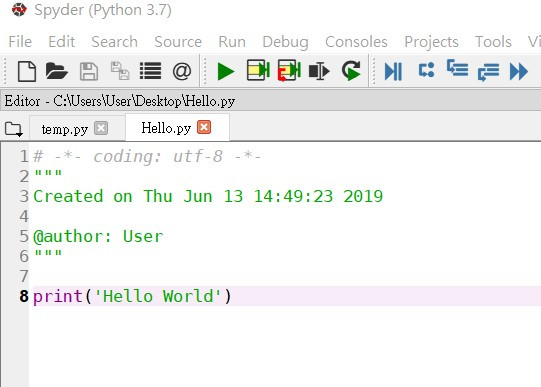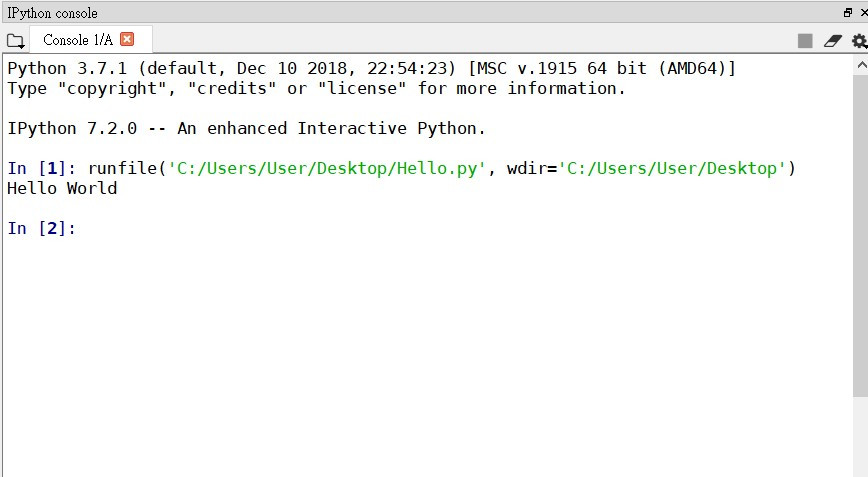#3

## 【Python 超入門】(3) 新手的疑惑- 程式? 我按計算機都算的比你快

【Python 超入門】(1) 心原一馬從零開始帶你學程式
【Python 超入門】(2) 心原一馬從零開始帶你認識spyder開發環境

# 程式碼的解說方式Hello World這幾個字，如下圖:``````print('Hello World')
``````

`Hello World`

# 情境-炎炎夏日正好眠?打工儲蓄去囉~

``````print(150*8*5*4)
``````

(注意，這是數學運算，不要用引號把150*8*5*4包起來)

`24000`
OK，那假設你暑假前一個月做的很好，老闆幫你加薪到時薪200，

``````print(200*8*5*4)
``````

# 變數-儲存資料的好幫手

python使用變數的規則很簡單，

``````hour_salary = 150
work_hour_per_day = 8
work_day_per_week = 5
print(hour_salary * work_hour_per_day * work_day_per_week * 4)
``````

``````hour_salary = 200
work_hour_per_day = 10
work_day_per_week = 5
print(hour_salary * work_hour_per_day * work_day_per_week * 4)
``````

# 程式比計算機方便的地方-「存檔」

month_fee=5000代表月花費5000元。

``````hour_salary = 200
work_hour_per_day = 10
work_day_per_week = 5
month_fee=5000
print(hour_salary * work_hour_per_day * work_day_per_week * 4 - month_fee)
``````

# 讓你的程式更好讀-「註解」的效用

``````hour_salary = 200       #設定時薪
work_hour_per_day = 10  #設定每天的工作時數
work_day_per_week = 5   #設定每週
month_fee = 5000        #設定每個月生活費
print(hour_salary * work_hour_per_day * work_day_per_week * 4 - month_fee) #計算每月存的錢
``````

# 附錄: 合法的變數名稱

• 變數名稱只能用英文字母、數字、_(底線)或中文字組成 (但建議不要用中文字幫變數取名)
• 英文字大小寫不一樣，例如: Name 和 name 是不同的變數名稱
• python內建函數不可以當作變數名稱 (例如: print)
• 變數不可以用阿拉伯數字當開頭，如 3a 是不合法的名字